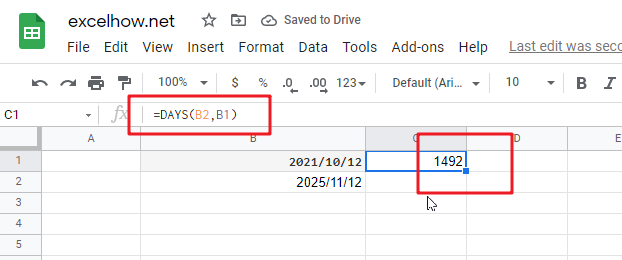This post will guide you how to use Google Sheets DAYS function with syntax and examples.

## Description

The Google Sheets DAYS  returns the number of days between two dates.

The DAYS function can be used to get the number of days between two given dates in google sheets. The purpose of this function is to get days between dates and its returned values is a number indicating days.

The DAYS function is a build-in function in Google Sheets and it is categorized as a Date function.

## Syntax

The syntax of the DAYS function is as below:

= DAYS (end_date,start_date)

Where the DAYS function arguments is:

• End_date -This is a required argument. The end of the date
• Start_date – this is a required argument. The start of the date

Note:

• Both two date arguments must be a valid date value, or it will return the #VALUE! Error.
• The DAYS function only works with whole numbers.
• If end dates and start dates are reversed, the DAYS function will return a negative number.
• The DAYS function will return all days between two dates. If you want to calculate all working days between dates, you can use the NETWORKDAYS function in google sheets.

## Google Sheets DAYS Function Examples

The below examples will show you how to use google sheets DAYS Function to return the number of days between end_date and start_date.

#1 =DAYS (B2,B1)Note: the above google sheets formula returns the number of days (1492) between the end date (2025/11/12) and start date (2021/10/12).